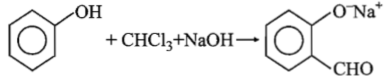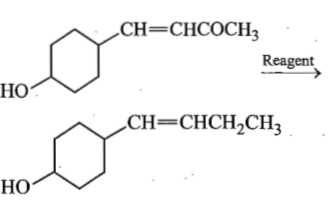In the reaction, Phenol $\underset{\mathrm{DIstillation}}{\overset{\mathrm{Zn}}{\to }}$(A) (B) $\underset{\mathrm{NaOH}\left(\mathrm{aq}\right)}{\overset{\mathrm{Zn}}{\to }}$(C)

The compounds (A),(B) and (C) are of the following:

(a) benzene, nitrobenzene and aniline

(b) benzene, dinitrobenzene and m-nitroaniline

(c) toluene, m-nitrobenzene and m-toluidine

(d) benzene, nitrobenzene and hydrazobenzene

Concept Questions :-

Phenols: Preparation, Properties, Acidic Nature of Phenol
High Yielding Test Series + Question Bank - NEET 2020

Difficulty Level:

Phenol on oxidation gives chloranil. The oxidant used is:

1. K2S2O8

2. KMnO4

3. KCIO3 + HCl

4. none of these

Concept Questions :-

Phenols: Preparation, Properties, Acidic Nature of Phenol
High Yielding Test Series + Question Bank - NEET 2020

Difficulty Level:

A characteristic group test for phenolic gp. is:

1. Liebermann's nitroso reaction
2. coupling with diazonium salt
3. aqueous FeCl3
4. all of the above

Concept Questions :-

Phenols: Preparation, Properties, Acidic Nature of Phenol
High Yielding Test Series + Question Bank - NEET 2020

Difficulty Level:

Phenol can be converted into salicylic acid by
(a) Etard's reaction
(b) Kolbe's reaction
(c) Reimer-Tiemann reaction
(d) both (b) and (c)

Concept Questions :-

Phenols: Preparation, Properties, Acidic Nature of Phenol
High Yielding Test Series + Question Bank - NEET 2020

Difficulty Level:

Resorcinol and conc. H2SO4 in presence of phthalic anhydride produce a compound which is:

1. a dye

2. an antiseptic

3. an indicator

4. a detergent

Concept Questions :-

Phenols: Preparation, Properties, Acidic Nature of Phenol
High Yielding Test Series + Question Bank - NEET 2020

Difficulty Level:

Phenol is weakly acidic but does not react with NaHCO3 like carboxylic acids hence:-

1. phenol is weaker than carbonic acid
2. phenol is stronger than carbonic acid
3. phenol is stronger than carboxylic acid
4. none of the above

Concept Questions :-

Phenols: Preparation, Properties, Acidic Nature of Phenol
High Yielding Test Series + Question Bank - NEET 2020

Difficulty Level:

An organic compound C7H8O is neither soluble in NaOH nor gives blue colour with FeCl3, is:

(a) C6H5.CH2OH                 (b) ${\mathrm{C}}_{6}{\mathrm{H}}_{4}{<}_{\mathrm{OH}}^{{\mathrm{CH}}_{3}}$

(c) C6H5.OCH3                   (d) none of these

Concept Questions :-

Ethers: Preparation, Physical & Chemical Properties, Uses
High Yielding Test Series + Question Bank - NEET 2020

Difficulty Level:The electrophile involved in the above reaction is:
1. dichloromethyl cation ($\stackrel{\oplus }{\mathrm{C}}{\mathrm{HCl}}_{2}$)
2. dichlorocarbene (:CCl2)
3. trichloromethyl anion ($\stackrel{⊝}{\mathrm{C}}{\mathrm{Cl}}_{3}$)
4. formyl cation ($\stackrel{\oplus }{\mathrm{C}}\mathrm{HO}$)

Concept Questions :-

Phenols: Preparation, Properties, Acidic Nature of Phenol
High Yielding Test Series + Question Bank - NEET 2020

Difficulty Level:

Which of the following reagents may be used to distinguish between phenol and benzoic acid?

(a) Aqueous NaOH

(b) Tollens reagent

(c) Molisch reagent

(d) Neutral FeCl3

Concept Questions :-

Phenols: Preparation, Properties, Acidic Nature of Phenol
High Yielding Test Series + Question Bank - NEET 2020

Difficulty Level:

In the given transformation, which of the following is the most appropriate reagent ?(a) Zn-Hg/HCl

(b) Na, Liq. NH3

(c) NaBH4

(d) NH2NH2,OH

Concept Questions :-

Alcohols: Preparation, Physical and Chemical Properties